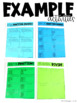# 5th Grade Math Journal Fractions Multiply and DivideSubject
Resource Type
File Type

PDF

(2 MB|48 pages)
Product Rating
4.0
(1 Rating)
Standards
Also included in:
1. ***This is a growing bundle. It includes 7 sets of journal activities now (out of the 9 total) and will continue to grow. If you purchase this bundle at a discount as it is growing, you will always be able to access it and the new files as they are added at no additional cost. This journal set cov
\$42.00
• Product Description
• StandardsNEW

This journal set covers multiplying and dividing fractions for fifth grade standards. There are 20 journal activities. (CC and TEKS) with answer keys.

Students will cut and paste the activities into a math journal. Each page contains the directions needed to complete the activity. These activities are meant to independent, however, they make terrific teaching tools and can be used any way that supports your teaching.

These activities provide a way for students to apply what they have learned while keeping a record of learning. I use these in my guided math rotations as a review and application.

Math journals can be used for the application and practice of learned material.

To see the style of the journal activities check out the preview file.

Aligned Guided Math Lessons for Whole Group and Small Group

Guided Math Fifth Grade Bundle for the Year

Thank you

Reagan Tunstall

Tunstall's Teaching Tidbits

Interpret division of a whole number by a unit fraction, and compute such quotients. For example, create a story context for 4 ÷ (1/5), and use a visual fraction model to show the quotient. Use the relationship between multiplication and division to explain that 4 ÷ (1/5) = 20 because 20 × (1/5) = 4.
Interpret division of a unit fraction by a non-zero whole number, and compute such quotients. For example, create a story context for (1/3) ÷ 4, and use a visual fraction model to show the quotient. Use the relationship between multiplication and division to explain that (1/3) ÷ 4 = 1/12 because (1/12) × 4 = 1/3.
Explaining why multiplying a given number by a fraction greater than 1 results in a product greater than the given number (recognizing multiplication by whole numbers greater than 1 as a familiar case); explaining why multiplying a given number by a fraction less than 1 results in a product smaller than the given number; and relating the principle of fraction equivalence 𝘢/𝘣 = (𝘯×𝘢)/(𝘯×𝘣) to the effect of multiplying 𝘢/𝘣 by 1.
Comparing the size of a product to the size of one factor on the basis of the size of the other factor, without performing the indicated multiplication.
Find the area of a rectangle with fractional side lengths by tiling it with unit squares of the appropriate unit fraction side lengths, and show that the area is the same as would be found by multiplying the side lengths. Multiply fractional side lengths to find areas of rectangles, and represent fraction products as rectangular areas.
Total Pages
48 pages
N/A
Teaching Duration
1 month
Report this Resource to TpT
Reported resources will be reviewed by our team. Report this resource to let us know if this resource violates TpT’s content guidelines.